### The MSTRUCT Model

Subsections:

In contrast to other modeling languages where the mean and covariance structures are implied from the specification of equations, paths, variable-factor relations, mean parameters, variance parameters, or covariance parameters, the MSTRUCT modeling language is supported in PROC CALIS for modeling mean and covariance structures directly.

A simple example for using the MSTRUCT modeling language is the testing of a covariance model with equal variances and covariances. Suppose that a variable was measured five times in an experiment. The covariance matrix of these five measurements is hypothesized to have the structure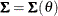where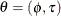and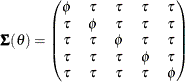For model structures that are hypothesized directly in the covariance matrix, the MSTRUCT modeling language is the most convenient to use. You can also use other general modeling languages such as LINEQS, PATH, or RAM to fit the same model structures, but the specification is less straightforward and more error-prone. For convenience, models that are specified using the MSTRUCT modeling language are called MSTRUCT models.

#### Model Matrices in the MSTRUCT Model

Suppose that there are p observed variables. The two model matrices, their names, their roles, and their dimensions are summarized in the following table.

Matrix

Name

Description

Dimensions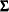_COV_ or _MSTRUCTCOV_

Structured covariance matrix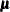_MEAN_ or _MSTRUCTMEAN_

Structured mean vector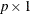#### Specification of the MSTRUCT Model

##### Specifying Variables

In the MSTRUCT statement, you specify the list of p manifest variables of interest in the VAR= list. For example, you specify v1v5 as the variables to be analyzed in your MSTRUCT model by this statement:

mstruct VAR= v1 v2 v3 v4 v5;


See the for details about the syntax.

The manifest variables in the VAR= list must be referenced in the input set. The number of variables in the VAR= list determines the dimensions of the _COV_ and the _MEAN_ matrices in the model. In addition, the order of variables determines the order of row and column variables in the model matrices.

##### Specifying Parameters in Model Matrices

Denote the parameter vector in the MSTRUCT model as. The dimension ofdepends on your hypothesized model. In the preceding example,contains two parameters inand. You can use the MATRIX statement to specify these parameters in the _COV_ matrix:

matrix _COV_ [1,1] = 5*phi,  /* phi for all diagonal elements */
[2, ] = tau,    /* tau for all off-diagonal elements */
[3, ] = 2*tau,
[4, ] = 3*tau,
[5, ] = 4*tau;


In this MATRIX statement, the five diagonal elements, starting from the [1,1] element of the covariance matrix, are fitted by the phi parameter. The specification 5*phi is a shorthand for specifying phi five times, once for each of the five diagonal elements in the covariance matrix. All other lower triangular elements are fitted by the tau parameter, as shown in the MATRIX statement. For example, with [3,] the elements starting from the first element of the third row of the _COV_ matrix are parameterized by the tau parameter. The specification 2*tau repeats the specification two times, meaning that the [3,1] and [3,2] elements are both fitted by the same parameter tau. Similarly, all lower triangular elements (not including the diagonal elements) of the _COV_ matrix are fitted by the tau parameter. The specification of the upper triangular elements (diagonal excluded) of the _COV_ matrix is not needed because the _COV_ matrix is symmetric. The specification in the lower triangular elements is transferred automatically to the upper triangular elements. See the for details about the syntax.

#### Default Parameters in the MSTRUCT Model

By using the MATRIX statements, you can specify either fixed values or free parameters (with or without initial values) for the elements in the _COV_ and _MEAN_ model matrices. If some or all elements are not specified, default parameters are applied to the MSTRUCT. There are two types of default parameters: one is free parameters; the other is fixed zeros. They are applied in different situations.

If you do not specify any elements of the _COV_ matrix with the MATRIX statement, all elements of the _COV_ matrix are free parameters by default. PROC CALIS names the default free parameters with the _Add prefix and a unique integer suffix. However, if you specify at least one fixed or free parameter of the _COV_ matrix with the MATRIX statement, then all other unspecified elements of the _COV_ matrix are fixed zeros by default.

If the mean structures are modeled, the same treatment applies to the _MEAN_ vector. That is, if you do not specify any elements of the _MEAN_ vector with the MATRIX statement, all elements of the _MEAN_ vector are free parameters by default. However, if you specify at least one fixed or free parameter of the _MEAN_ vector with the MATRIX statement, then all other unspecified elements of the _MEAN_ vector are fixed zeros by default.

##### How and Why the Default Parameters Are Treated Differently in the MSTRUCT Model

Notice that the default parameter treatment of the MSTRUCT model is quite different from other types of models such as FACTOR, LINEQS, LISMOD, RAM, or PATH. For these models, unspecified variances and covariances among exogenous variables are all free parameters by default. However, for the MSTRUCT model, either default free parameters or fixed zeros are generated depending on whether at least one element of the covariance matrix is specified. The reason for this different treatment is that you fit the covariance structure directly by using the MSTRUCT modeling language. Hence, in an MSTRUCT model there is no information regarding the functional relationships among the variables that indicates whether the variables are exogenous or endogenous in the model. Hence, PROC CALIS cannot assume default free parameters based on the exogenous or endogenous variable types.

Because of this special default parameter treatment, when fitting an MSTRUCT model you must make sure that each diagonal element in your _COV_ matrix is set as a free, constrained, or fixed parameter, in accordance with your theoretical model. If you specify some elements in the model matrix but omit the specification of other diagonal elements, the default fixed zero variances would lead to a nonpositive definite _COV_ model matrix, making the model fitting problematic.